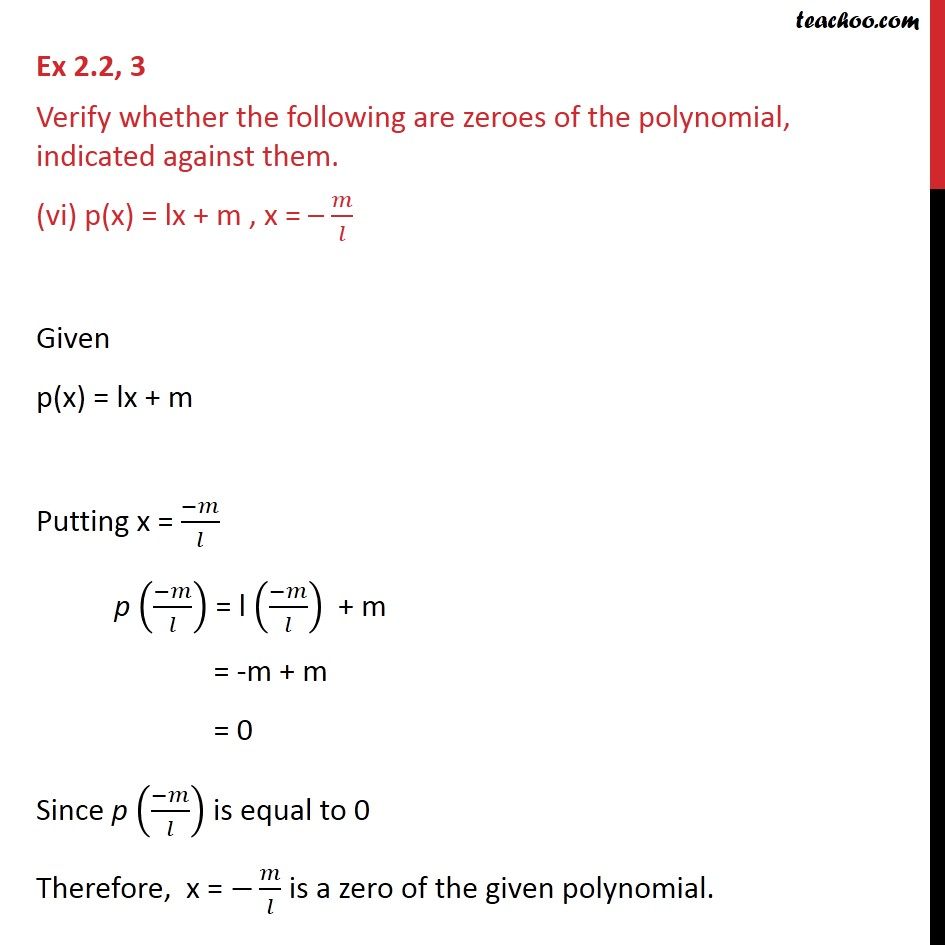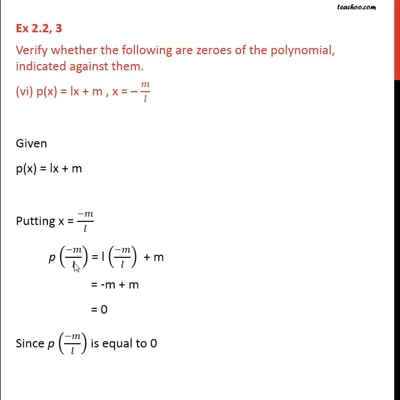Ex 2.2

Chapter 2 Class 9 Polynomials
Serial order wiseThis video is only available for Teachoo black users

Introducing your new favourite teacher - Teachoo Black, at only ₹83 per month

### Transcript

Ex 2.2, 3 Verify whether the following are zeroes of the polynomial, indicated against them. (vi) p(x) = lx + m , x = – 𝑚/𝑙 Given p(x) = lx + m Putting x = (−𝑚)/𝑙 p ((−𝑚)/𝑙) = l ((−𝑚)/𝑙) + m = -m + m = 0 Since p ((−𝑚)/𝑙) is equal to 0 Therefore, x = −𝑚/𝑙 is a zero of the given polynomial.